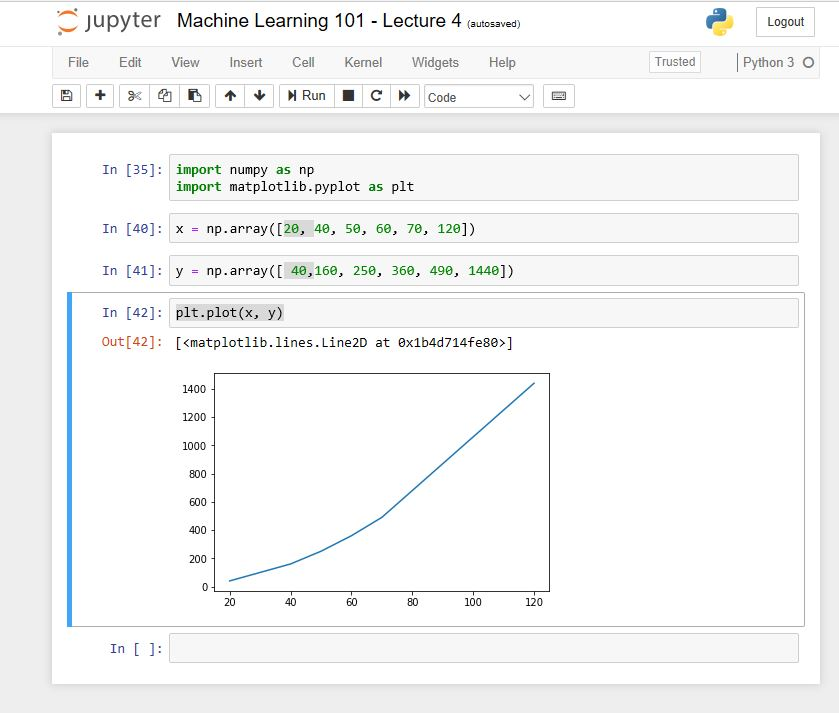# Machine Learning 101 – Simple Regression Problem

This is Lecture 4 of the Machine Learning 101. It follows from Lecture 3.  In this lecture, we would solve some regression problems. So brace up!

First, What is Regression?

Simply put, regression is a method used to find relationship between two or more variables.

Problem 1: Marketing Ads and Profit

You have been hired by a departmental store as a Machine Learning expert. Your task is to predict how much profit they would make is they spent \$20.00 on advertising. What if they spend additional \$120.00 on advertising, how much profit would they make. To help you, they provide you with a history of how spendings on ads and corresponding profit.

The record is given below:

 Year Spending (\$) Profit (\$) 2000 40 160 2005 50 250 2010 60 360 2016 70 490 2019 20 ? 2020 120 ?

Solution 1: By Inspection

We first examine the given data.

For example, in the year 2000, \$40.00 yielded a profit of \$160.00. This seems like 40 x 40 = 1600 (but not exactly).

What of (40 x 40) / 10? That would give us 1600/10 = 160.

If we apply it to the year 2005, we would have (50 x 50) / 10 = 250.

It seems we have it!

Now we need to find the formula that relates spending  to profit. Say we need to find y = f(x)

Let’s use the variable x to be spending

Also, let’s use the variable y to be profit

Let’s also write x = {40, 50, 60, 70, 20, 120)

y = {160, 250, 360, 490, y5, y6}

From out analysis, we found out that y = f(x) = x2 /10

Now it would be easy to find y5 and y6

y5 = f(20) = 202 / 10 = 400/10 = 40

y6 = f(120) = 1202 / 10 = 14400/10 = 1440

Therefore, if the supermarket spends \$20.00 in 2019, then they would make a profit of \$40.00

Similarly, if they spend \$120.00 in 2020, they would make a profit of \$1,440.00

Congrats!. You have solved a problem using Machine Learning

A Few Notes

What we just solved is actually a polynomial regression problem. This is because variable x is not linear in function we derived (we have x2).

Other regression types include linear regression and logistic regression. We would cover these in subsequent classes.

In the next lecture we would solve some linear regression problem. Then we would also do the same using python.

Practicals: How to Plot in Python

To conclude this class, I will show you how to plot this data in Python. I’m sure you have Jupyter Notebook installed.

Just to remind you: Jupyter notebook provide environment where you write Machine Learning programs using Python syntax.

The screenshot of the complete program is shown below. Ensure to do it yourself.

Notice that we rearranged the data and placed (20, 40)  in the first position:#### kindsonthegenius

Kindson Munonye is currently completing his doctoral program in Software Engineering in Budapest University of Technology and Economics

View all posts by kindsonthegenius →